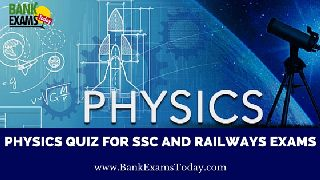New Students Offer - Use Code HELLO

# Physics Quiz for SSC and Railways Exams (Set- 2)Today we are presenting you a 10 question set - 2 on Physics which is very important for your upcoming SSC CGL 2016 and RRB NTPC 2016. Try to solve it.

## Physics Quiz for SSC and Railways Exams

1. Which of the following measurements is not a unit of distance?
a) Ammeter
b) Cubit
c) Parsec
d) Angstrom

2. Pure water freezes at what temperature?
a) 47 F
b) 32 F
c) 0 F
d) 19 F

3. Which one of the following remains constant while throwing a ball upward?
a) Displacement
b) Kinectic energy
c) Acceleration
d) Velocity

4. Which law is also called law of inertia?
a) Newton first law
b) Newton second law
c) Newton third law
d) All of above

5. Solar cell converts
a) Sound energy into electrical energy
b) Electrical energy into mechanical energy
c) Solar energy into electrical energy
d) Electrical energy into light energy

6. Who discovered Diode Bulb?
a) Henry Becquerel
b) Sir J.S. Fleming
c) Lee De Forest
d) Archemedes

7. Siphon will fail to work if
a) both its limbs are of unequal length
b) the temperature of the liquids in the two vessels are the same
c) the level of the liquid in the two vessels are at the same height
d) the densities of the liquid in the two vessels are equal

8. Force of attraction between the molecules of different substances is called
a) Surface tension
b) Cohensive force
d) None of above

9. What is newton third law of motion?
a) Every body maintains its initial state rest or motion unless no external force is applied)
b) The rate of change in momentum of a body is directly proportional to the applied force on the body and takes place in the direction of force.
c) To every action there is equal and opposite reaction.
d) None of above.

10. Which is true?
a) In a static liquid at same horizontal level, pressure is more at centre
b) In a static liquid at same horizontal level, pressure is less at centre.
c) In a static liquid at same horizontal level, pressure is same at all points
d) In a static liquid at same horizontal level, pressure is different at all points

1. a) Ammeter
2. b) 32 F
3. c) Acceleration
4. a) Newton first law
5. c) Solar energy into electrical energy
6. b) Sir J.S. Fleming
7. c) the level of the liquid in the two vessels are at the same height
9. c) To every action there is equal and opposite reaction.
10. c) In a static liquid at same horizontal level, pressure is same at all points

Thanks.